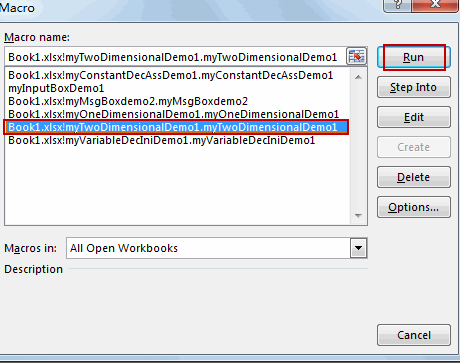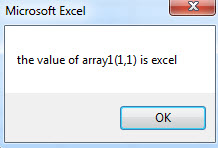# Excel VBA Array

This post will guide you how to create one-dimensional array and two-dimensional array in MS excel VBA.

Array is a specific variable and it can hold more than one value in a single variable.

### VBA One-dimensional Array Declaration

Array declaration can be used the following three syntax:

1. Dim array1()
2. Dim array2(1 to 5)
3. array3 = Array(“one”, “two”, “three”)

### VBA One-dimensional Array assignation

This section will describe that how to assign value to VBA array.

```Dim array2(1 To 5) As String
array2(1) = "excel"
array2(2) = "word"
array2(3) = "access"
array2(4) = "ppt"
array2(5) = "oneNote"```

#### Example1:  VBA One-dimensional Array  example

1# open visual Basic Editor, then insert a module and name as :myOneDimensionalDemo1

2# enter into the below VBA codes in code window.

```Sub myOneDimensionalDemo1()
Dim array2(1 To 5) As String
array2(1) = "excel"
array2(2) = "word"
array2(3) = "access"
array2(4) = "ppt"
array2(5) = "oneNote"
MsgBox "the value of array2(1) is " & array2(1)
End Sub```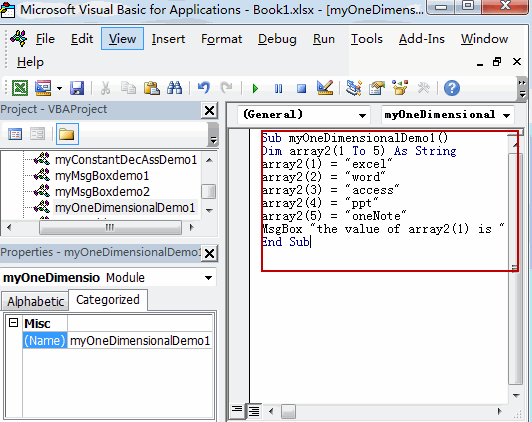3# back to workbook and run the above macro.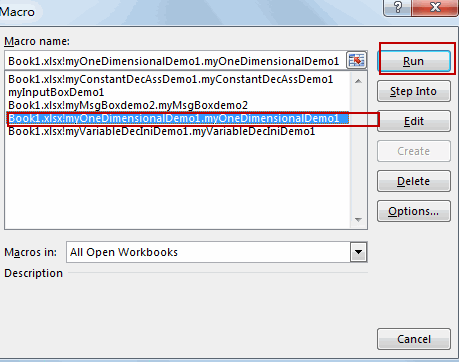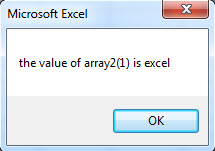### VBA two-dimensional Array Declaration

If you want to declare a string two-dimensional array, just refer to the below lines:

Dim array1(2,2) as String

Or

Dim array1(1 to 2, 1 to 2) as String

This Array declaration will declare with 2 rows and 2 columns.

### VBA Two-dimensional Array assignation

This section will describe that how to assign value to VBA two-dimensional array.

```Dim array1(1 to 2, 1 to 2) as String
Array1(1,1) = "excel"
Array1(1,2) = "word"
Array1(2,1) = "access"
Array1(2,2) = "ppt"```

#### Example2:  VBA two-dimensional Array  example

1# open visual Basic Editor, then insert a module and name as :myTwoDimensionalDemo1

2# enter into the below VBA codes in code window.

```Sub myTwoDimensionalDemo1()
Dim array1(1 To 2, 1 To 2) As String
array1(1, 1) = "excel"
array1(1, 2) = "word"
array1(2, 1) = "access"
array1(2, 2) = "ppt"
MsgBox "the value of array1(1,1) is " & array1(1, 1)
End Sub```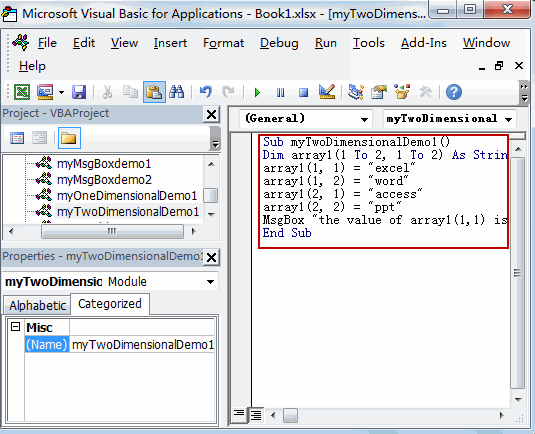3# back to workbook and run the above macro.